STAT 380

Problems: Assignment 2

1.
page 85, number 25, page 85, number 26 and page 87, number 40.

2.
page 146, number 8.

3.
Suppose X and Y are independent Geometric(p) random variables. In other words for non-negative integers j and k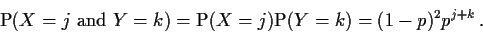(a)
Let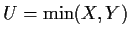,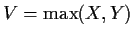and W=V-U. Express the event U=j and W=k in terms of X and Y.

(b)
Compute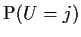and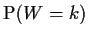and prove that the event U=j and the event W=k are independent. You will have to be careful of the case k=0.

(c)
A computer is waiting for two flags to be set. Both flags start out not set. For each flag the conditional probability that the flag is set next cycle given it is not yet set is 0.5. The two flags operate independently. How many cycles should you expect to wait before both flags are set including the cycle on which the last flag becomes set?

4.
page 145, number 5.

5.
page 146, number 11.

6.
page 146, number 15.

7.
page 150, number 33.

Richard Lockhart
2000-09-18